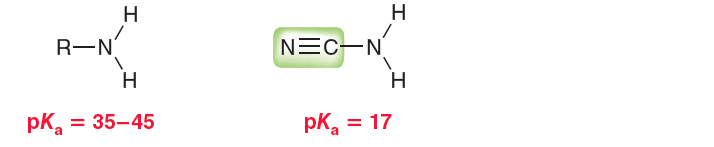# Problem: Most common amines (RNH 2) exhibit pKa values between 35 and 45. R represents the rest of the compound (generally carbon and hydrogen atoms). However, when R is a cyano group, the pKa is found to be drastically lower: (a) Explain why the presence of the cyano group so drastically impacts the pKa.

###### FREE Expert Solution
90% (208 ratings)
###### Problem Details

Most common amines (RNH 2) exhibit pKa values between 35 and 45. R represents the rest of the compound (generally carbon and hydrogen atoms). However, when R is a cyano group, the pKa is found to be drastically lower:

(a) Explain why the presence of the cyano group so drastically impacts the pKa.Frequently Asked Questions

What scientific concept do you need to know in order to solve this problem?

Our tutors have indicated that to solve this problem you will need to apply the Ranking Acidity concept. You can view video lessons to learn Ranking Acidity. Or if you need more Ranking Acidity practice, you can also practice Ranking Acidity practice problems.

What is the difficulty of this problem?

Our tutors rated the difficulty ofMost common amines (RNH 2) exhibit pKa values between 35 and...as high difficulty.

How long does this problem take to solve?

Our expert Organic tutor, Jonathan took undefined 51 seconds to solve this problem. You can follow their steps in the video explanation above.

What professor is this problem relevant for?

Based on our data, we think this problem is relevant for Professor Nguyen's class at SAC.

What textbook is this problem found in?

Our data indicates that this problem or a close variation was asked in Organic Chemistry - Klein 1st Edition. You can also practice Organic Chemistry - Klein 1st Edition practice problems.